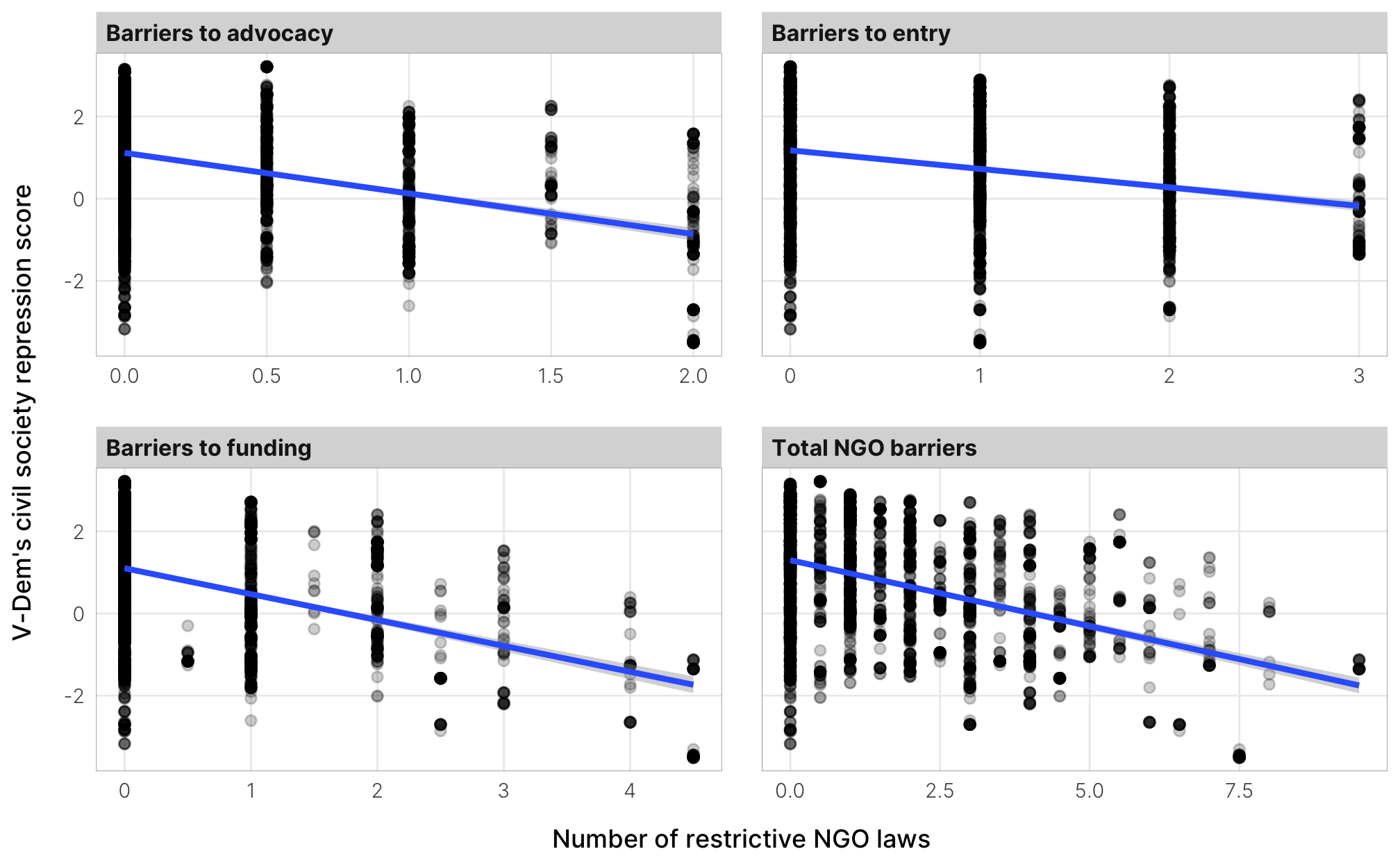library(tidyverse)
library(targets)
library(brms)
library(broom)
library(tidybayes)
library(sf)
library(scico)
library(colorspace)
library(scales)
library(patchwork)
library(ggtext)
library(here)

CHAINS <- 4
ITER <- 2000
WARMUP <- 1000
BAYES_SEED <- 1234
options(mc.cores = parallel::detectCores())  # Use all cores

# Generated via random.org
set.seed(9936)

# Need to use this withr thing because tar_read() and tar_load() need to see the
# _targets folder in the current directory, but this .Rmd file is in a subfolder
withr::with_dir(here::here(), {

})

# Civil society restrictions over time

things_to_map <- canary %>%
filter(year %in% c(1993, 2013)) %>%
select(country, year, iso3, barriers_total, PTS_factor, v2csreprss) %>%
pivot_wider(names_from = year, values_from = c(barriers_total, PTS_factor, v2csreprss)) %>%
mutate(new_laws = barriers_total_2013 - barriers_total_1993,
new_laws = ifelse(new_laws <= 0, 0, new_laws),
new_laws = ceiling(new_laws),
new_laws_cat = ifelse(new_laws >= 5, "5+", as.character(new_laws))) %>%
diff_csrepress_trunc = case_when(
diff_csrepress >= 2 ~ 2,
diff_csrepress <= -2 ~ -2,
TRUE ~ diff_csrepress
)) %>%
pct_change_csrepress_trunc = case_when(
pct_change_csrepress >= 5 ~ 5,
pct_change_csrepress <= -5 ~ -5,
TRUE ~ pct_change_csrepress
))

map_data <- world_map %>%
left_join(things_to_map, by = c("ADM0_A3_IS" = "iso3"))

## Legislation

map_ngo_laws <- ggplot() +
geom_sf(data = map_data, aes(fill = factor(new_laws_cat)),
color = "black", size = 0.1) +
coord_sf(crs = st_crs("ESRI:54030"), datum = NA) +  # Robinson
scale_fill_manual(values = seq_gradient_pal("#FFFFFF", "#CF4446")(seq(0, 1, length.out = 6)),
na.value = "grey95", breaks = c(as.character(0:4), "5+")) +
# scale_fill_manual(values = scico(7, palette = "devon", direction = -1),
#                   na.value = "grey80", breaks = 0:6) +
guides(fill = guide_legend(nrow = 1, label.position = "bottom",
keywidth = unit(1, units = "lines"),
keyheight = unit(0.6, units = "lines"))) +
labs(fill = "Restrictive NGO laws passed between 1993 and 2013") +
theme_ngo() +
theme(panel.border = element_blank(),
legend.box.margin = margin(t = 5),
legend.spacing.x = unit(0, "pt"),
legend.title = element_textbox_simple(halign = 1,
lineheight = 1.3,
minwidth = unit(2, "lines"),
maxwidth = unit(10, "lines"),
map_ngo_laws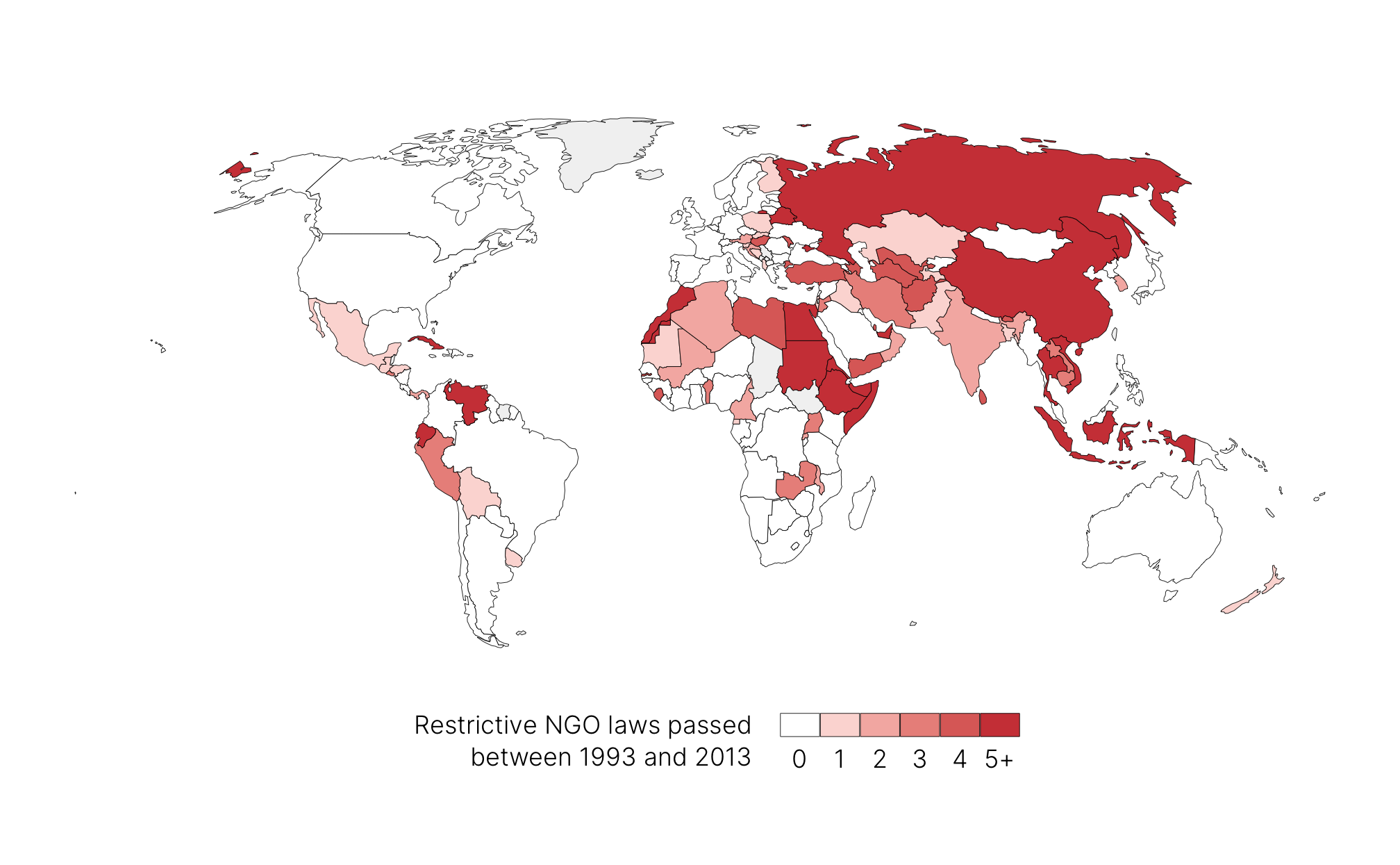## Civil society repression

map_cs_repression <- ggplot() +
geom_sf(data = map_data, aes(fill = diff_csrepress_trunc),
color = "black", size = 0.1) +
coord_sf(crs = st_crs("ESRI:54030"), datum = NA) +  # Robinson
scale_fill_scico(palette = "vik", direction = -1,
limits = c(-1, 1) * max(abs(map_data\$diff_csrepress_trunc), na.rm = TRUE),
breaks = seq(-4, 4, 1), na.value = "grey80") +
guides(fill = guide_colorbar(reverse = FALSE, ticks = FALSE,
barwidth = unit(7, units = "lines"),
barheight = unit(0.5, units = "lines"))) +
labs(fill = "Change in V-Dem civil society repression between 1990 and 2013") +
theme_ngo() +
theme(panel.border = element_blank(),
legend.box.margin = margin(t = 5),
legend.title = element_textbox_simple(halign = 1,
lineheight = 1.3,
minwidth = unit(2, "lines"),
maxwidth = unit(12, "lines"),
map_cs_repression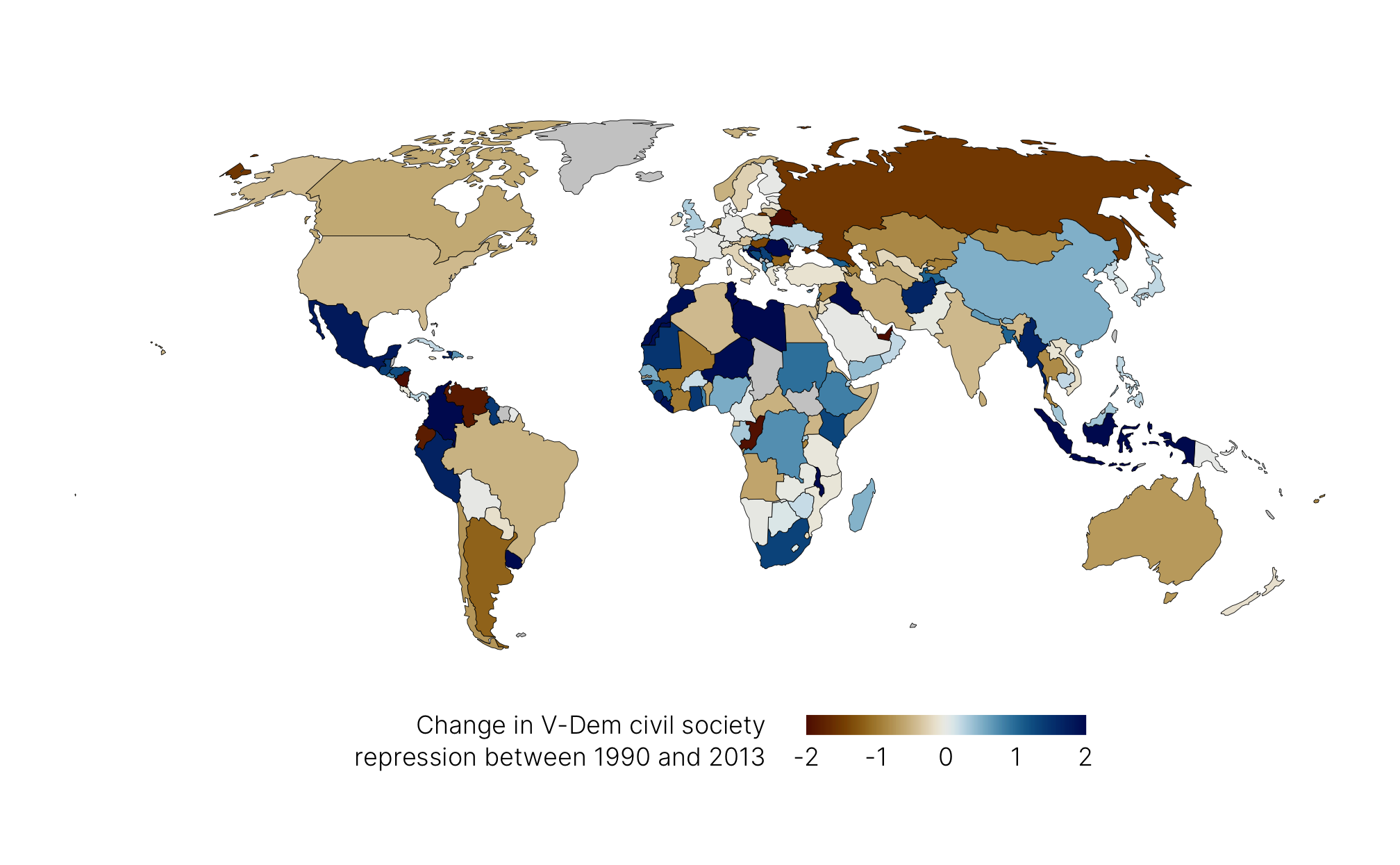# PTS and civil society restrictions

## V-Dem civil society repression and the PTS

diff_cs_pts <- brm(
bf(v2csreprss ~ 0 + PTS_factor, sigma ~ 0 + PTS_factor),
family = student,
data = filter(canary, year == 2010) %>% select(v2csreprss, PTS_factor),
prior = c(
# Set group mean prior
set_prior("normal(0, 1)", class = "b", lb = -4, ub = 4),
# Set group variance priors. We keep the less informative cauchy(0, 1).
set_prior("cauchy(0, 1)", class = "b", dpar = "sigma"),
set_prior("exponential(1.0/29)", class = "nu")
),
chains = CHAINS, iter = ITER, warmup = WARMUP, seed = BAYES_SEED
)
diff_cs_pts_summary <- diff_cs_pts %>%
gather_draws(b_PTS.*, regex = TRUE) %>%
median_hdci(.width = 0.95) %>%
to_broom_names()

diff_cs_pts_draws <- diff_cs_pts %>%
gather_draws(b_PTS.*, regex = TRUE) %>%
ungroup() %>%
mutate(.variable = str_replace(.variable, "b_PTS_factorLevel", "Level ")) %>%
mutate(pts_level = fct_rev(.variable))

diff_cs_pts_posterior <- canary %>%
filter(year == 2010) %>%
modelr::data_grid(PTS_factor) %>%
add_epred_draws(diff_cs_pts, seed = BAYES_SEED)
ggplot(diff_cs_pts_draws, aes(y = pts_level, x = .value, fill = pts_level)) +
stat_halfeye() +
scale_x_reverse() +
scale_fill_viridis_d(option = "inferno", begin = 0.2, end = 0.9, guide = "none") +
labs(x = "Civil society repression", y = "Political Terror Scale (PTS)") +
theme_ngo()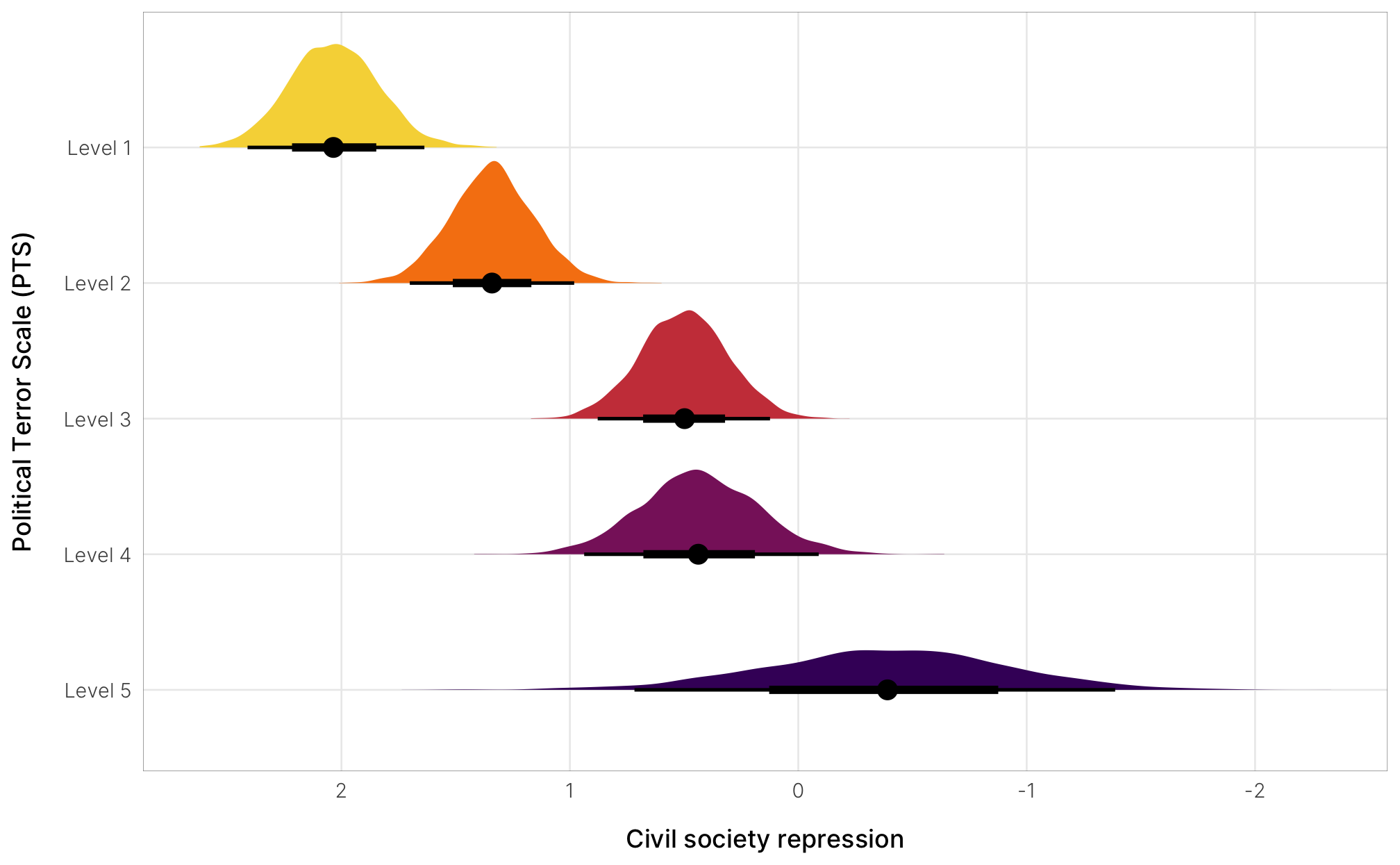ggplot(diff_cs_pts_posterior, aes(x = .epred, y = fct_rev(PTS_factor), color = fct_rev(PTS_factor))) +
stat_dots(quantiles = 100, shape = 19) +
stat_pointinterval(aes(point_colour = after_scale(colorspace::darken(color, 0.65)),
interval_colour = after_scale(colorspace::darken(color, 0.35)))) +
scale_x_reverse() +
scale_color_viridis_d(option = "inferno", begin = 0.2, end = 0.9, guide = "none") +
labs(x = "Civil society repression", y = "Political Terror Scale (PTS)",
caption = "Each • represents an equally likely outcome from the posterior distribution") +
theme_ngo()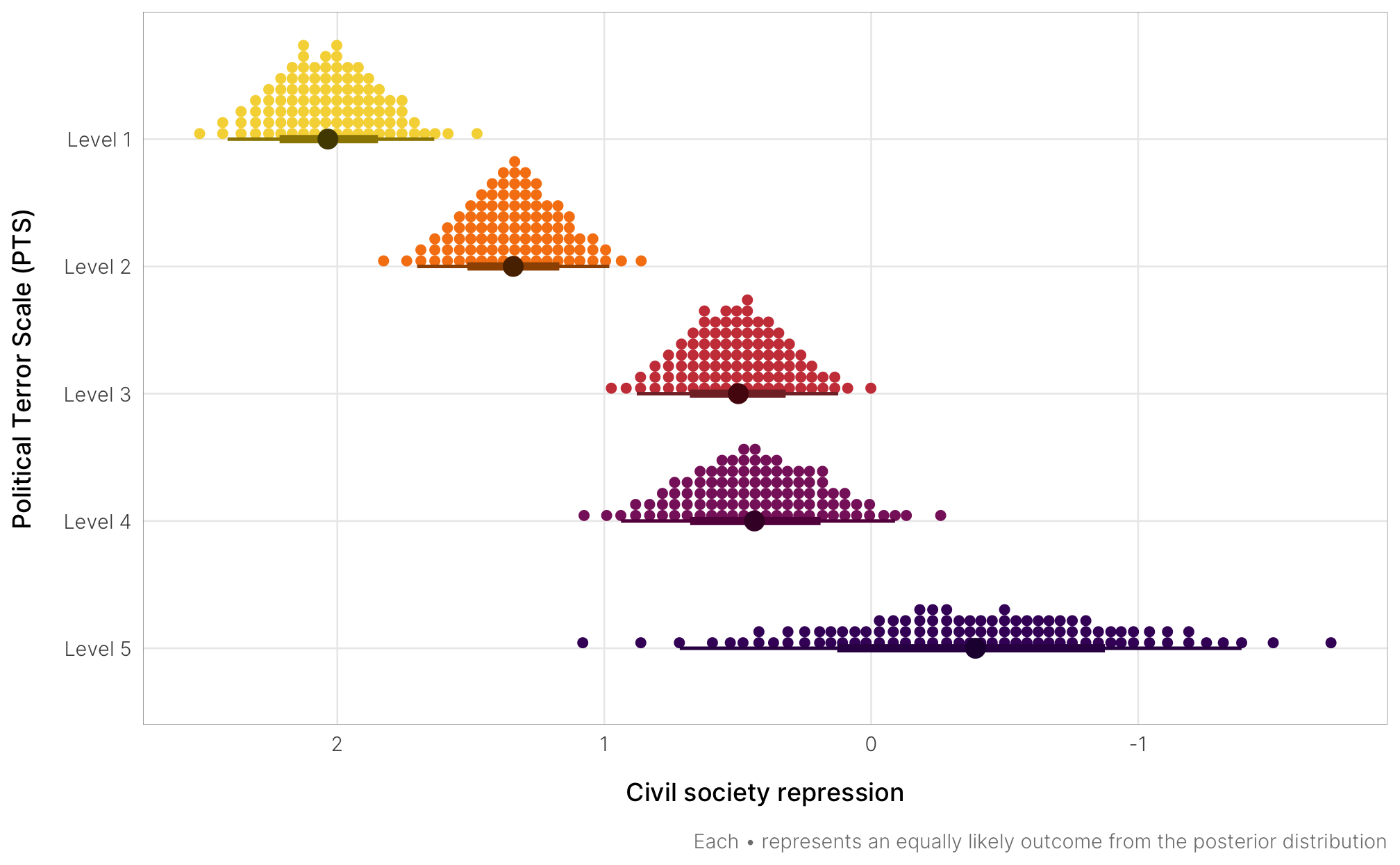## Chaudhry NGO regulations and V-Dem’s v2csreprss

canary_barriers <- canary %>%
pivot_longer(cols = c(advocacy, entry, funding, barriers_total),
names_to = "barrier", values_to = "count") %>%
entry = "Barriers to entry",
funding = "Barriers to funding",
barriers_total = "Total NGO barriers"))

ggplot(canary_barriers, aes(x = count, y = v2csreprss)) +
geom_point(alpha = 0.2) +
geom_smooth(method = "lm") +
labs(x = "Number of restrictive NGO laws",
y = "V-Dem's civil society repression score") +
facet_wrap(vars(barrier), scales = "free_x") +
theme_ngo()